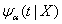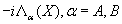NONLINEAR PHENOMENA IN COMPLEX SYSTEMS
An Interdisciplinary Journal

2006, Vol.9, No.1, pp.75-80

Master Equation with Non-Poissonian Statistics and Finite Duration of Collisions .
M. F. Gelin and A. P. Blokhin

Generalized master equation has been derived for the probability density function P(X,t) within the following assumptions: (i) a time realization of the stochastic process X(t) can be represented as a sequence of intervals of free motion (event A) interrupted by collisions (event B); (ii) durations of the events A and B are governed by arbitrary distributions; (iii) the time evolution of X(t) during the events A and B is described by the time-independent Liouville operators. The approach generalizes the standard Markovian master equations to non-instantaneous collisions and non-Poissonian collision statistics.
Key words: generalized master equation, non-poissonian collision statistics, non-instantaneous collisions

Full text:  Acrobat PDF  (104KB)

Copyright © Nonlinear Phenomena in Complex Systems. Last updated: April 25, 2006